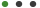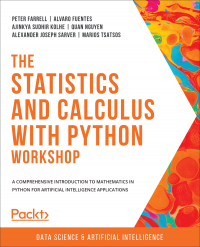# The Statistics and Calculus with python Workshop

A comprehensive introduction to mathematics in Python for artificial intelligence applicationsPeter FarrellPackt PublishingLanguage - EnglishUpdated on Oct, 2020Development,Data Science and AI ML,PythonThis eBook includes

Formats : PDF, EPUB, MOBI (Downlodable)

Pages : 740

ISBN : 9781800208360

Language : English

### Book description

With examples and activities that help you achieve real results, applying calculus and statistical methods relevant to advanced data science has never been so easy

## Key Features

• Discover how most programmers use the main Python libraries when performing statistics with Python
• Use descriptive statistics and visualizations to answer business and scientific questions
• Solve complicated calculus problems, such as arc length and solids of revolution using derivatives and integrals

## Book Description

Are you looking to start developing artificial intelligence applications? Do you need a refresher on key mathematical concepts? Full of engaging practical exercises, The Statistics and Calculus with Python Workshop will show you how to apply your understanding of advanced mathematics in the context of Python.

The book begins by giving you a high-level overview of the libraries you'll use while performing statistics with Python. As you progress, you'll perform various mathematical tasks using the Python programming language, such as solving algebraic functions with Python starting with basic functions, and then working through transformations and solving equations. Later chapters in the book will cover statistics and calculus concepts and how to use them to solve problems and gain useful insights. Finally, you'll study differential equations with an emphasis on numerical methods and learn about algorithms that directly calculate values of functions.

By the end of this book, you’ll have learned how to apply essential statistics and calculus concepts to develop robust Python applications that solve business challenges.

## What you will learn

• Get to grips with the fundamental mathematical functions in Python
• Perform calculations on tabular datasets using pandas
• Understand the differences between polynomials, rational functions, exponential functions, and trigonometric functions
• Use algebra techniques for solving systems of equations
• Solve real-world problems with probability
• Solve optimization problems with derivatives and integrals

## Who this book is for

If you are a Python programmer who wants to develop intelligent solutions that solve challenging business problems, then this book is for you. To better grasp the concepts explained in this book, you must have a thorough understanding of advanced mathematical concepts, such as Markov chains, Euler's formula, and Runge-Kutta methods as the book only explains how these techniques and concepts can be implemented in Python.## Author DetailsPackt Publishing

Founded in 2004 in Birmingham, UK, Packt's mission is to help the world put software to work in new ways, through the delivery of effective learning and information services to IT professionals.

Working towards that vision, we have published over 6,500 books and videos so far, providing IT professionals with the actionable knowledge they need to get the job done - whether that's specific learning on an emerging technology or optimizing key skills in more established tools.

As part of our mission, we have also awarded over \$1,000,000 through our Open Source Project Royalty scheme, helping numerous projects become household names along the way.